Thermodynamics and Propulsion

# 18.6 Muddiest Points on Chapter 18

MP 18..1   For an electric heated strip embedded between two layers, what would the temperature distribution be if the two side temperatures were not equal?

If the two temperatures on the outer surfaces of the composite layer wereand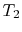, the heat flux from the two sides of the electrical heater would be different, but the sum of the two heat fluxes would still be equal to the heat generated per unit area and unit time. In fact heat could be coming into one side of the heater if one of the temperatures were high enough. (Sketch this out and prove it to yourself.) The temperature distribution would be linear from the heater temperature to the two surface temperatures. If this example is not clear, please come and see me.

MP 18..2   Why did you change the variable and write the derivative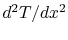as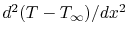in the equation for heat transfer in the fin?

The heat balance and derivation of the equation for temperature Equation (18.8) is given in Section 18.2 of the notes. This isIt is not necessary to change variables, and one could solve (18.8) as is or in the formHowever, since: (1) the reference temperature is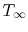, and what is of interest is the difference, (2) making the substitution results in a simpler form of the equation to be solved, and (3) the derivative ofis the same as the derivative of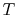, I found it convenient to put it the equation in the form of (18.9),MP 18..3   What types of devices use heat transfer fins?

A number of types of heat exchangers use fins. Examples of the use of fins you may have seen are cooling fins on motorcycle engine heads, cooling fins on electric power transformers, or cooling fins on air conditioners.

MP 18..4   Why did the Stegosaurus have cooling fins? Could the stegosaurus have heating fins?''

My knowledge of this issue extends only to reading about it in the text by Lienhard (see reference in notes). The journal article referenced in that text is: Farlow, J. O., Thompson, C. V., and Rosner, D.E., Plates of the Dinosaur Stegosaurus: Forced Convection Heat Loss Fins?'', Science, vol. 192, no. 4244, 1976, pp. 1123-1125 and cover.

MP 18..5   In equation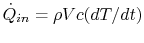(18.15), what is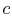?is the specific heat for a unit mass. We don't have to differentiate between the two specific heats for a solid because the volume changes are very small, unlike a gas.

MP 18..6   In the lumped parameter transient heat transfer problem, does a high density slow down'' heat transfer?

It doesn't. The high density slows down the rate at which the object changes temperature; high density means more heat capacity.''

UnifiedTP